# Geometry and 2D Shapes: Comparing Triangles

In this worksheet, students identify right-angled, isosceles, equilateral and scalene triangles.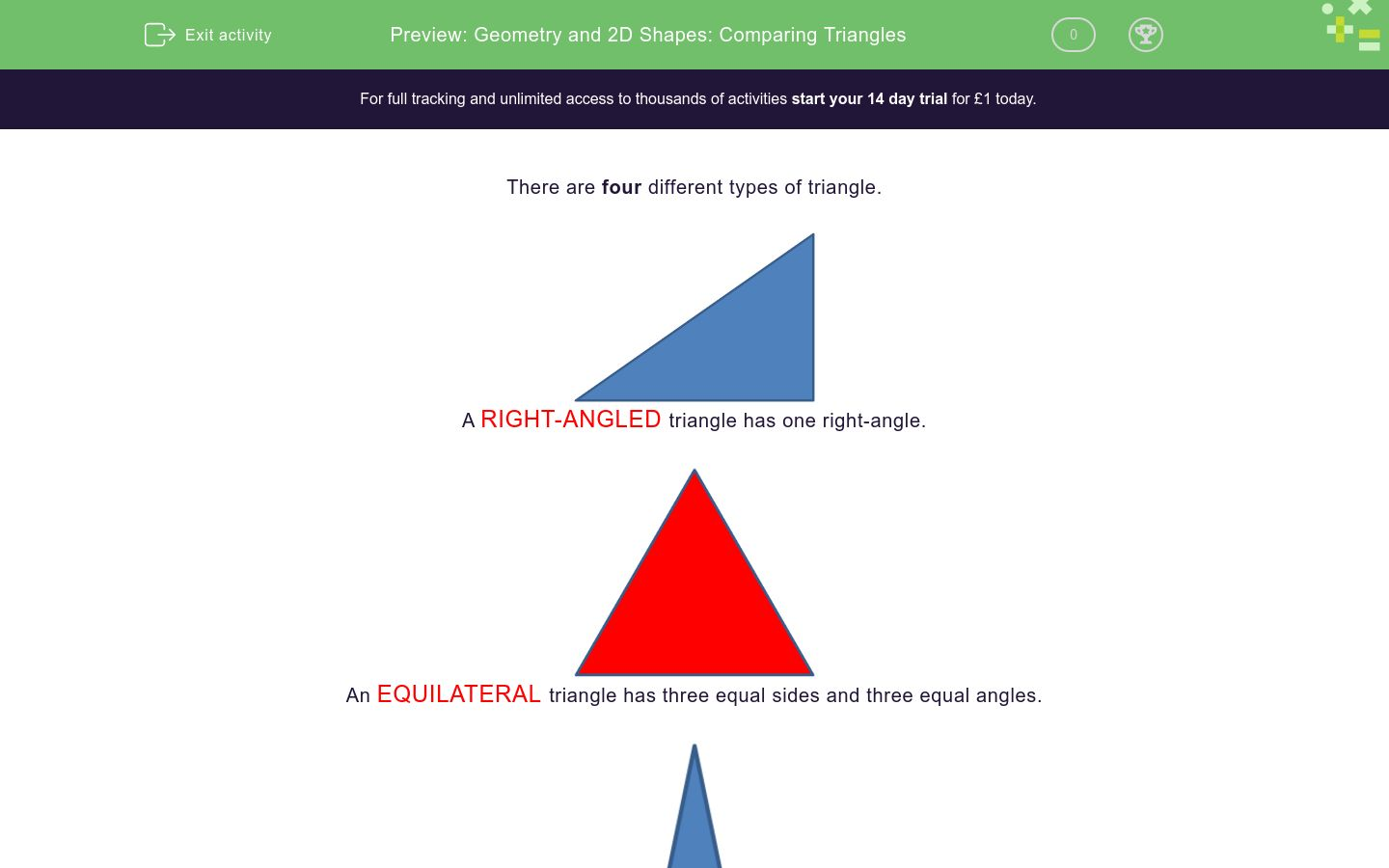Key stage:  KS 2

Curriculum topic:   Geometry: Properties of Shapes

Curriculum subtopic:   Compare Geometric Shapes

Difficulty level:\$appendString = '&new=1'; \$_SESSION['guest_aId'] = rand(); \$urlToRedirect = FRAMEWORK_PATH . 'preview/worksheet/introduction?aId=' . \$_SESSION['guest_aId'] . '&wId=' . \$wsData['id'] . '&usertype=guest' . \$appendString; ?>

### QUESTION 1 of 10

There are four different types of triangle.RIGHT-ANGLED triangle has one right-angle.An EQUILATERAL triangle has three equal sides and three equal angles.An ISOSCELES triangle has two equal sides and two equal angles.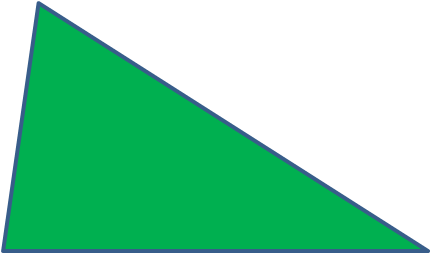SCALENE triangle has three different sides and three different angles.

Which sort of triangle is this?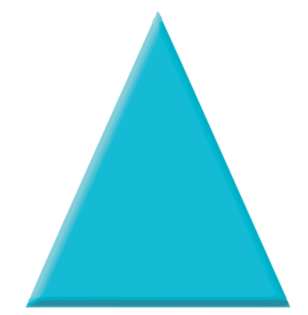Equilateral

Isosceles

Right-Angled

Scalene

Which sort of triangle is this?Equilateral

Isosceles

Right-Angled

Scalene

Which sort of triangle is this?Equilateral

Isosceles

Right-Angled

Scalene

Which sort of triangle is this?Equilateral

Isosceles

Right-Angled

Scalene

Which sort of triangle is this?Equilateral

Isosceles

Right-Angled

Scalene

Which sort of triangle is this?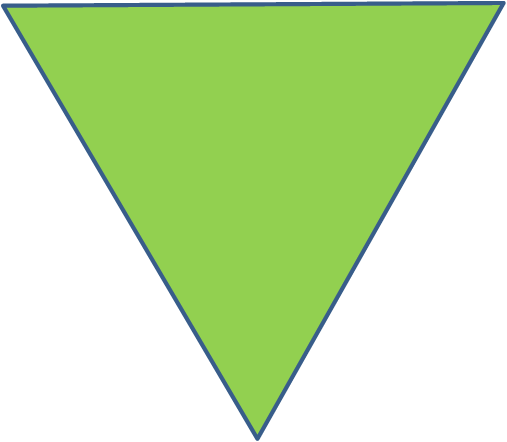Equilateral

Isosceles

Right-Angled

Scalene

Which sort of triangle is this?Equilateral

Isosceles

Right-Angled

Scalene

Which sort of triangle is this?Equilateral

Isosceles

Right-Angled

Scalene

Which sort of triangle is this?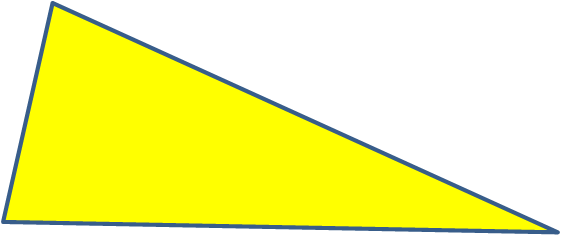Equilateral

Isosceles

Right-Angled

Scalene

Which sort of triangle is this?Equilateral

Isosceles

Right-Angled

Scalene

• Question 1

Which sort of triangle is this?Isosceles
EDDIE SAYS
An isosceles triangle has two equal angles.
• Question 2

Which sort of triangle is this?Scalene
EDDIE SAYS
A scalene triangle has 3 different angles.
• Question 3

Which sort of triangle is this?Right-Angled
EDDIE SAYS
A right-angled triangle has a 90 degree angle.
• Question 4

Which sort of triangle is this?Equilateral
EDDIE SAYS
An equilateral triangle has 3 equal angles.
• Question 5

Which sort of triangle is this?Scalene
EDDIE SAYS
A scalene triangle has three different angles.
• Question 6

Which sort of triangle is this?Equilateral
EDDIE SAYS
An equilateral triangle has three equal angles.
• Question 7

Which sort of triangle is this?Isosceles
EDDIE SAYS
An isosceles triangle has two equal angles.
• Question 8

Which sort of triangle is this?Right-Angled
EDDIE SAYS
A right-angled triangle has a 90 degree angle.
• Question 9

Which sort of triangle is this?Scalene
EDDIE SAYS
A scalene triangle has three different angles.
• Question 10

Which sort of triangle is this?Right-Angled
EDDIE SAYS
A right-angled triangle has a 90 degree angle.
---- OR ----

Sign up for a £1 trial so you can track and measure your child's progress on this activity.

### What is EdPlace?

We're your National Curriculum aligned online education content provider helping each child succeed in English, maths and science from year 1 to GCSE. With an EdPlace account you’ll be able to track and measure progress, helping each child achieve their best. We build confidence and attainment by personalising each child’s learning at a level that suits them.

Get started